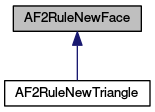MeshKit  1.0
AF2RuleNewFace Class Reference

`#include <AF2RuleNewFace.hpp>`

Inheritance diagram for AF2RuleNewFace:[legend]

List of all members.

## Public Member Functions

virtual ~AF2RuleNewFace ()
virtual unsigned int getNumVertices () const =0
Get the number of vertices that the element this rule would create would have.
virtual unsigned int getVertexIndex (unsigned int vtxNum) const =0
Get the index of one of the vertices of the element that the rule would create.

## Detailed Description

Definition at line 10 of file AF2RuleNewFace.hpp.

## Constructor & Destructor Documentation

 virtual ~AF2RuleNewFace ( ) ` [inline, virtual]`

Definition at line 14 of file AF2RuleNewFace.hpp.

## Member Function Documentation

 virtual unsigned int getNumVertices ( ) const` [pure virtual]`

Get the number of vertices that the element this rule would create would have.

Returns:
the number of vertices that the element would have

Implemented in AF2RuleNewTriangle.

 virtual unsigned int getVertexIndex ( unsigned int vtxNum ) const` [pure virtual]`

Get the index of one of the vertices of the element that the rule would create.

Indices returned by this method index into the list of vertices associated with the rule, i.e., a list consisting of a list of the rule's existing vertices followed by a list of the new vertices that the rule would create. The numbering of the vertices of the face begins with 0, so the valid arguments to this method are 0 through n - 1, where n is the value returned from getNumVertices().

Parameters:
 vtxNum the number of the vertex for which to get the index
Returns:
the index in the list of the rule vertices of vertex number vtxNum of the element

Implemented in AF2RuleNewTriangle.

The documentation for this class was generated from the following file: